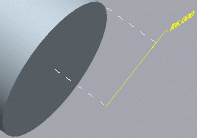Example: Z-extension Lines
The following examples are of Z-extension lines in linear, angular, diameter dimensions, and radius dimensions.
Z-extension Lines in a Linear Dimension
In the following figure, the Z-extension line is attached to the edge of the surface at a distance closest to the dimension witness line.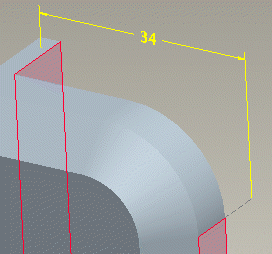Z-extension Lines in an Angular Dimension
The following figure shows Z-extension lines for angular dimensions.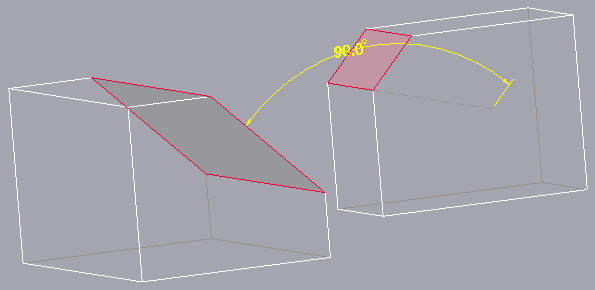Z-extension Lines in a Diameter Dimension
For diameter dimensions with two arrowheads, the Z-extension line extends from each arrowhead as shown in the following figure.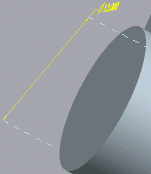Z-extension Lines in a Radius Dimension
For a radius dimension with a single arrowhead (no extensions), a single Z-extension line extends from the reference to the dimension arrowhead as shown in the following figure.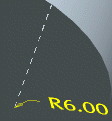For a radius dimension shown with flipped arrows with a leader line extension to the center, there are two Z-extension lines. One line extends to the reference, while the other extends to the center of the reference.